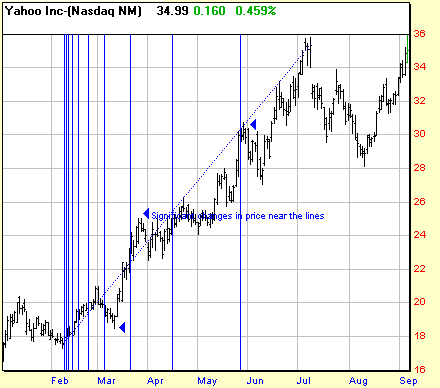# Fibonacci Time Zones

Fibonacci time zones are composed by dividing a chart with vertical lines spaced apart in a ratio adhering to the Fibonacci number sequence (1, 1, 2, 3, 5, 8, 13, etc.). The interpretation of Fibonacci Time Zones involves looking for significant price movement near the vertical lines. Also known as Fibonacci Time Series.

Related Articles: Trading with Fibonacci NumbersChart courtesy of Prophet Financial Systems (www.prophet.net)